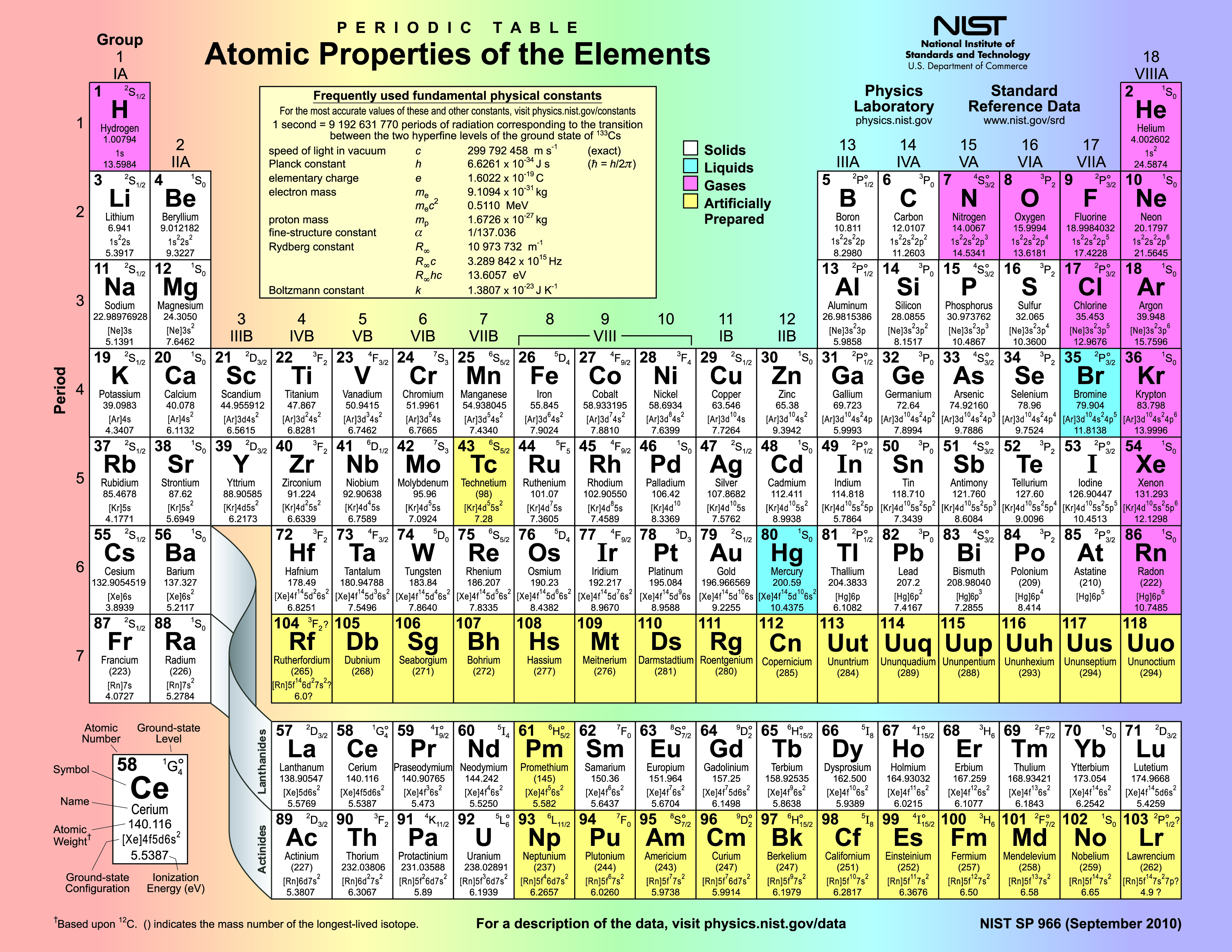Universal Constants: A New Foundation of Measurement    >   Standardize - Achieve Precision

# The Mole

The mole is the unit for the amount of substance—how many atoms, molecules, ions, electrons, or any other particle or specified group of particles. Learn more about the mole and practice some calculations here.

The mole is essentially “a measure of stuff.” It helps a chemist describe the amount of matter (number of atoms) in a reaction in a very specific way. Lots of atoms! For example, 1.0 milligram piece of copper wire contains about 9.5 x 1018 [a]atoms. That is about the same number of atoms as there are grains of sand on all the beaches in the world. Clearly, even small amounts of substances have very large numbers of atoms. Scientists need a way to describe these very large numbers to predict reactions, energy exchange, and other phenomena. They do it with the mole.

The mole is simply the number of particles (atoms or ions) in a substance. A mole of water (18 grams) or a mole of carbon-12 (0.012 kg) each contain exactly 6.02214076 × atoms. This huge number is usually referred to as NA,[b] or Avogadro’s number, after Italian physicist Amedeo Avogadro (1776–1856). The mole enables estimates of tiny amounts of reagent in a reaction, making extreme precision possible.

Using a unit that refers to a number is not such a strange concept if you think about it. Have you ever gotten a dozen ears of corn? The word dozen describes how many ears of corn you have, which is the number 12. In the same way, the mole stands for the number 6.02214076 × 1023[c]. The particles measured with the mole include atoms, molecules, ions, groups of ions described by the formula of an ionic compound, and electrons. It can also be used to describe the strength of a solution, when one mole of solute is dissolved in a liter of water.

In a more technical sense, the old definition relied on other constants like the kilogram. A mole was the amount of substance that contained as many elementary entities as there were atoms in 0.012 kilograms of the most common form of carbon, known as carbon-12. Remember that platinum-iridium cylinder locked up in a special vault outside Paris, France. So if the cylinder changed (even the smallest bit) then the atoms in the mole might change too.

When the kilogram was redefined in 2018 (in terms of Planck’s Constant, which you will read about in Lesson 3), the mole was also redefined. We no longer think of a quantity equal to 12 g of carbon, but to the number of atoms in the sample—that big number for a tiny quantity.

The effort to redefine the kilogram and the mole were interrelated. For years, metricians at NIST and other labs worked to create “perfect” 1 kg spheres of silicon-28. Instead of a chunk of carbon-12 atoms, they use a uniform crystal of silicon-28, carefully machined into spheres with a mass of 1 kg. It took several measurements of these spheres to confirm the number with an uncertainty of less than 20 parts per billion.

### Molar Mass

The molar mass is the mass of 1 mole of particles. Because different particles have different masses, their molar masses are different. For carbon and for all other elements, the molar mass is the same numerical value as the atomic mass of one atom. The only difference is in the units: molar mass is measured in grams per mole (g/mol), whereas atomic mass is measured in atomic mass units (amu).

Molar mass allows you to easily convert from numbers of atoms/molecules to number of moles to number of grams in a chemical equation. For example, in a chemical equation, coefficients can refer to atoms/ions/molecules or to moles. Using molar mass, moles can be converted to grams.

In the months preceding the redefinition of the kilogram, some metrologists argued that Avogadro’s number might be a better constant than Planck’s, and cited the work done on that “perfect” silicon sphere to support their position. But Planck’s constant had several advantages. It was a more direct way to measure. (You’ll see that as you explore the Kibble Balance in Lesson 3.) And it was directly linked to other units used in electronics.

### Using the Mole

In Activity - Absolutely Hot you use a 0.025 M solution of plaster of Paris. One way to understand the unit is to use it in some problems. Follow along with these, then return to the activity to answer the Analysis questions.1. You have 223.380 grams of iron. How many atoms are in your sample?

Work backwards to determine the calculations you need to make. To find the number of atoms of iron, you have to find the number of moles of iron. To find the number of moles of iron, you have to find the molar mass of iron.

Find the molar mass of iron. Look at iron on the periodic table to find the mass of a single iron atom. That value is 55.845 amu. The molar mass of iron will have the same numerical value as the atomic mass (55.845) but with units of grams per mole. So, the molar mass of iron is 55.845 grams/mole (or g/mol).

Find the number of moles of iron in 223.380 grams of iron. Invert the molar mass and use it as a conversion factor: 1 mole/55.845 grams x 223.380 grams = 4.000 moles.

Find the number of atoms of iron in 4.000 moles of iron. Use the conversion factor 6.02214076 x1023 atoms/ mole: 6.02214076 x1023 atoms/mole x 4.000 moles = 24.089 x1023 atoms, which is 2.4089 x1024 atoms in scientific notation.[d]

2. You have 1.000 mole of water (H2O[e]). How much mass does it have?